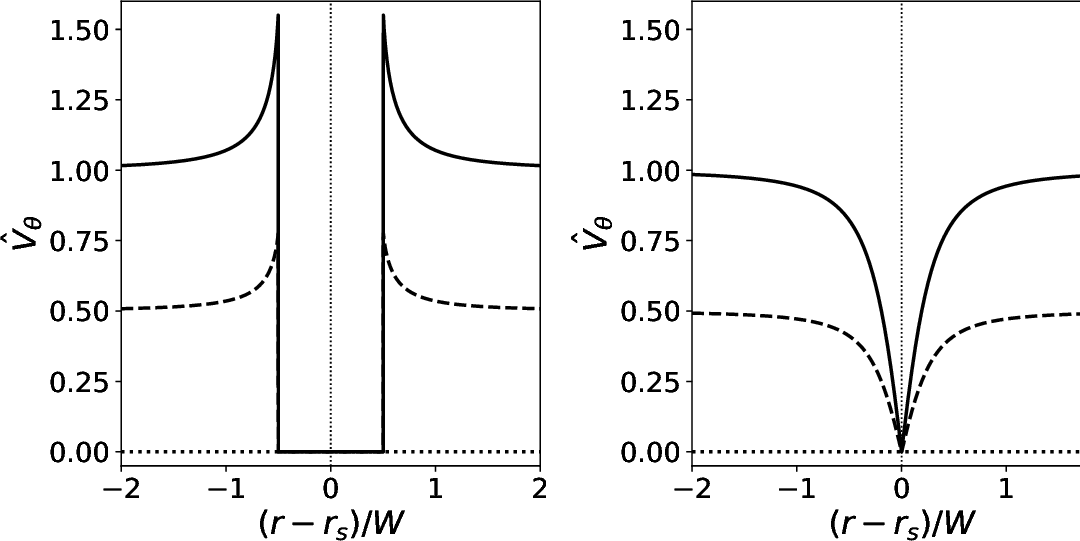# Saturated Island Width

Equation (9.5) gives the impression that if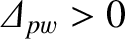then the width of the magnetic island chain grows without limit. In fact, this is not the case. Instead, the width of the island chain eventually stops growing, and the tearing mode attains a saturated steady state. In order to model this effect, it is necessary to perform the asymptotic matching between the inner and outer regions (see Sections 3.8 and 4.1) to higher order, taking into account the finite width of the island chain [5,12,15,18].

For the case of an island chain that is sufficiently wide to flatten the plasma pressure within its magnetic separatrix , the appropriate saturation theory is given in Hastie, et alia . According to this theory,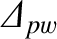in Equation (9.5) must be replaced by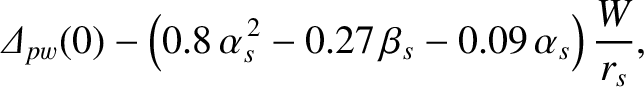(9.15)

where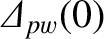denotes the zero-island-width, perfect-wall, tearing stability index,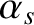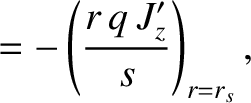(9.16)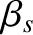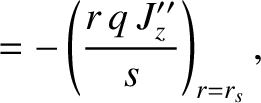(9.17)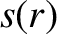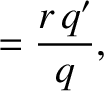(9.18)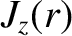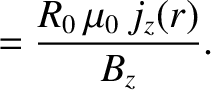(9.19)

Here,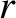is a radial coordinate in cylindrical geometry,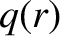the safety-factor profile (see Section 3.2),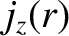the equilibrium toroidal current density,the simulated major radius of the plasma,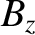the equilibrium toroidal magnetic field-strength, anddenotes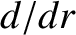.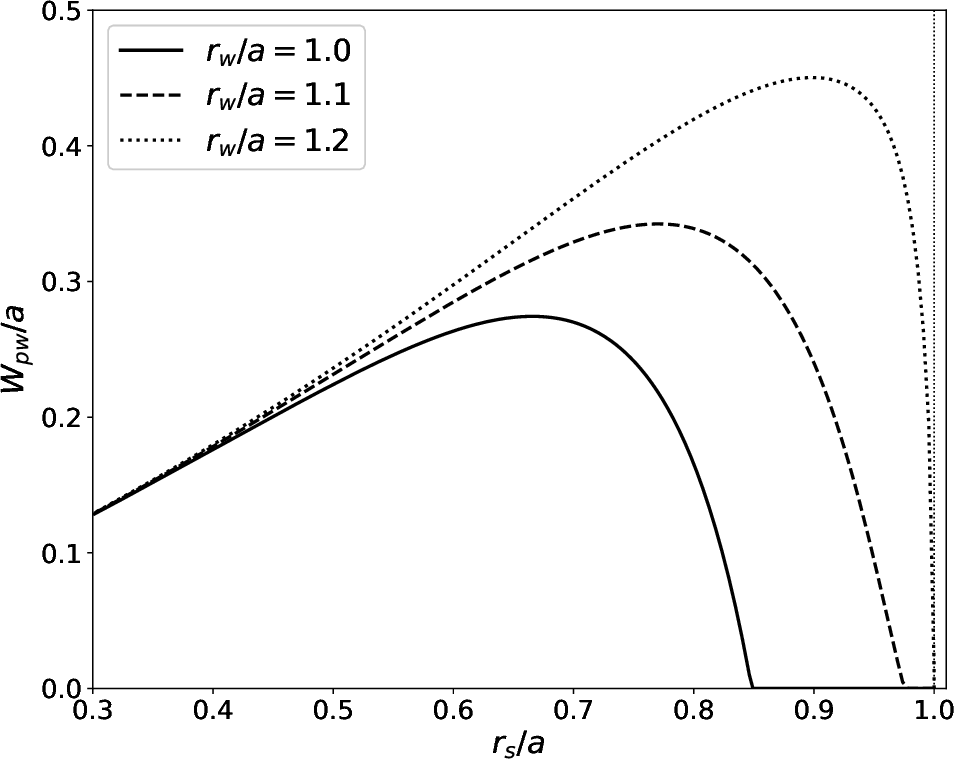Equations (9.5) and (9.15) can be combined to give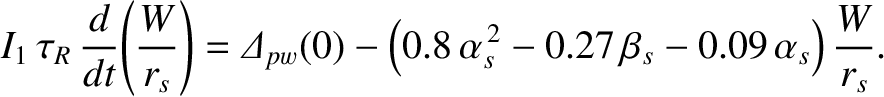(9.20)

If we define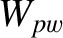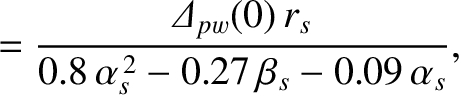(9.21)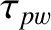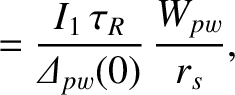(9.22)

then Equation (9.20) reduces to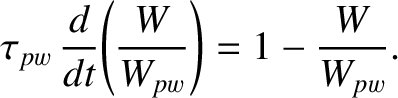(9.23)

The previous equation can be solved to give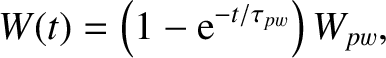(9.24)

assuming that the island width is zero at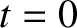. It follows that the width of the island chain does not grow without limit, but instead eventually attains the saturated value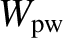. Moreover, the time required to achieve saturation,, is of order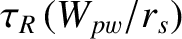.

Figure 9.2 shows the saturated island width of an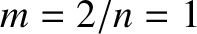tearing mode, which is generally the most unstable tearing mode in a conventional tokamak plasma , in a so-called “Wesson” equilibrium  characterized by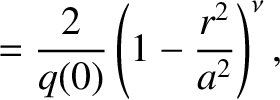(9.25)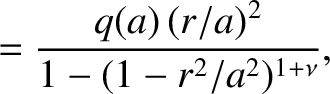(9.26)

where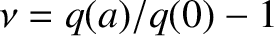. Here,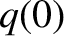and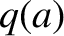are the safety-factor values at the magnetic axis,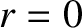, and the plasma boundary,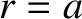, respectively. In the calculation shown in Figure 9.2,is determined by solving the cylindrical tearing mode equation, subject to suitable boundary conditions, as described in Section 3.8. Moreover,is given the plausible value 0.8 , whileis varied in order to shift the position of the rational surface within the plasma. It can be seen that if the rational surface lies well inside the plasma boundary then the 2/1 island chain saturates at relatively small width (i.e.,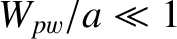). On the other hand, if the rational surface lies close to the plasma boundary then the island chain saturates at a much higher width. However, the presence of a close-fitting perfectly conducting wall can mitigate this effect to some extent.

Given that the minor radius,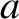, of a tokamak fusion reactor is a few meters (see Table 1.2), whereas the typical linear layer width is a few millimeters (see Table 6.2), it is clear from Figure 9.2 that the saturated island width of an unstable tearing mode in a tokamak fusion reactor is bound to be very much larger than the linear layer width. Thus, we conclude that the time evolution of an unstable tearing mode in such a reactor will only be governed by linear theory for a comparatively short period of time after its onset, its subsequent time evolution being governed by nonlinear theory. Furthermore, it will take a time of order the resistive diffusion time,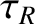, for the mode to attain its final saturated amplitude.

Note, finally, that a saturated magnetic island chain degrades the overall energy confinement properties of a tokamak plasma because it flattens the pressure profile within its magnetic separatrix (see Chapter 8 and Section 14.18) . Moreover, the degree of degradation is roughly proportional to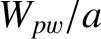.